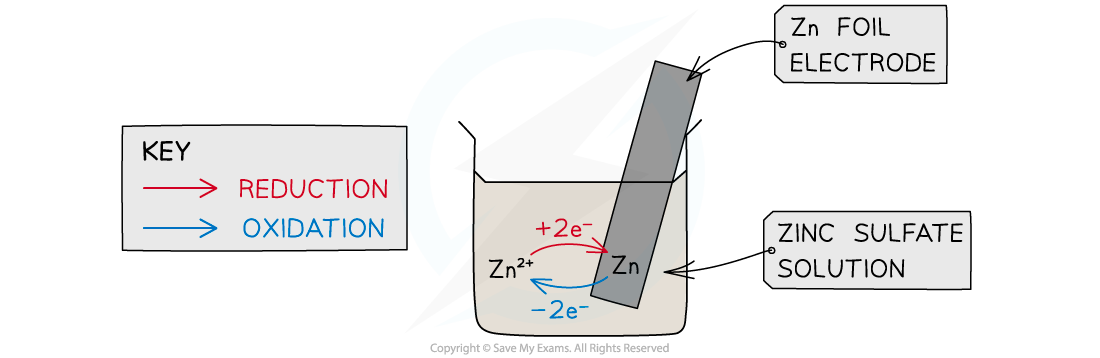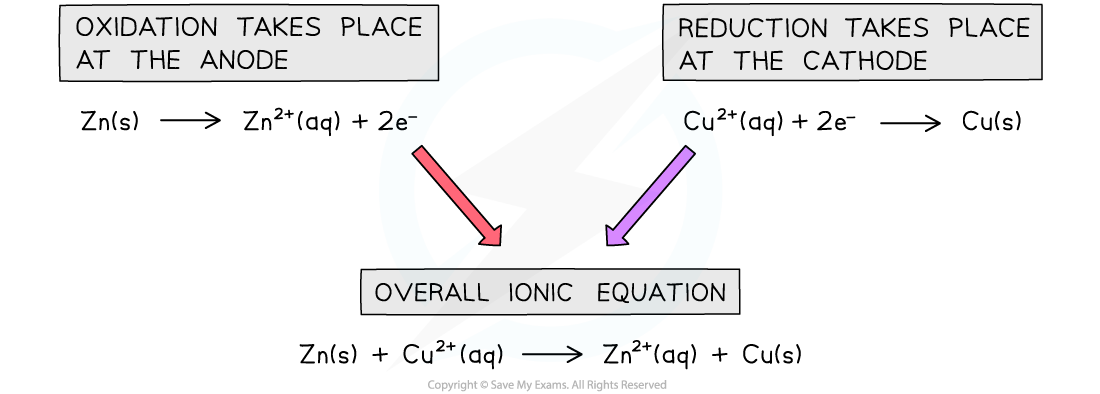# IB DP Chemistry: SL复习笔记9.1.9 Voltaic Cells

### Voltaic Cells

• Voltaic (or Galvanic) cells generate electricity from spontaneous redox reactions
• For example:

Zn (s)  + CuSO4 (aq)→ Cu (s)  + ZnSO4 (aq)

• Instead of electrons being transferred directly from the zinc to the copper ions a cell is built which separates the two redox processes
• Each part of the cell is called a half cell
• If a rod of metal is dipped into a solution of its own ions, an equilibrium is set up
• For example:

Zn (s)  ⇌  Zn2+ (aq) + 2eWhen a metal is dipped into a solution contains its ions an equilibrium is established between the metal and it ions

• This is a half cell and the strip of metal is an electrode
• The position of the equilibrium determines the potential difference between the metal strip and the solution of metal
• The Zn atoms on the rod can deposit two electrons on the rod and move into solution as Zn2+ ions:Zn(s) ⇌ Zn2+(aq) + 2e
• This process would result in an accumulation of negative charge on the zinc rod
• Alternatively, the Zn2+ ions in solution could accept two electrons from the rod and move onto the rod to become Zn atoms:Zn2+(aq) + 2e ⇌ Zn(s)
• This process would result in an accumulation of positive charge on the zinc rod
• In both cases, a potential difference is set up between the rod and the solution
• This is known as an electrode potential
• A similar electrode potential is set up if a copper rod is immersed in a solution containing copper ions (eg CuSO4), due to the following processes:

Cu2+(aq) + 2e ⇌ Cu(s)   - reduction (rod becomes positive)

Cu(s) ⇌ Cu2+(aq) + 2e   - oxidation (rod becomes negative)

• Note that a chemical reaction is not taking place - there is simply a potential difference between the rod and the solution
• The potential difference will depend on
• the nature of the ions in solution
• the concentration of the ions in solution
• the type of electrode used
• the temperature

#### Creating an emf

• If two different electrodes are connected, the potential difference between the two electrodes will cause a current to flow between them. Thus an electromotive force (emf) is established and the system can generate electrical energy
• A typical electrochemical cell can be made by combining a zinc electrode in a solution of zinc sulphate with a copper electrode in a solution of copper sulphateThe zinc-copper voltaic cell ( also known as the Daniell Cell)

• The circuit must be completed by allowing ions to flow from one solution to the other
• This is achieved by means of a salt bridge - often a piece of filter paper saturated with a solution of an inert electrolyte such as KNO3(aq)
• The e.m.f can be measured using a voltmeter
• Voltmeters have a high resistance so that they do not divert much current from the main circuit
• The combination of two electrodes in this way is known as an electrochemical or voltaic cell, and can be used to generate electricity
• The positive electrode or cathode is the one which most favours reduction
• In this case it is the copper electrode which is positive
• The negative electrode or anode is the one which most favours oxidation
• In this case it is the zinc electrode which is negative
• Thus electrons flow from the zinc electrode to the copper electrodeIonic equations for the Daniell Cell

• To maintain charge in the half cells:
• anions flow to the negative half cell to replace the negative charge of the electrons
• cations flow to the positive half cell since there is a surplus positive charge from the metals becoming cations in the negative half cell
• The sulphate ions flow through the salt bridge from the Cu2+(aq) solution to the Zn2+(aq) solution, to complete the circuit and compensate for the reduced Cu2+ concentration and increased Zn2+ concentration
• The overall cell reaction including spectator ions can thus be written as follows:

Zn (s)  + CuSO4 (aq)→ Cu (s)  + ZnSO4 (aq)

• The external connection must be made of a metallic wire in order to allow electrons to flow
• The salt bridge must be made of an aqueous electrolyte to allow ions to flow
• By allowing two chemical reagents to be connected electrically, but not chemically, a reaction can only take place if the electrons flow externally
• The chemical energy is thus converted into electrical energy.

#### Exam Tip

Students often confuse the redox process that take place in voltaic cells and electrolytic cells. An easy way to remember is the phrase RED CATS:REDuction takes place at the CAThode !